Function Repository Resource:

# RicciCurvature

Compute the components of the Ricci curvature for a metric

Contributed by: Wolfram Staff (original content by Alfred Gray)
 ResourceFunction["RicciCurvature"][m,{u,v}] computes the components of the Ricci curvature for a metric.

## Details and Options

The Ricci curvature quantifies the local deviation of the geometry of a metric tensor from ordinary Euclidean space.

## Examples

### Basic Examples (5)

 In:=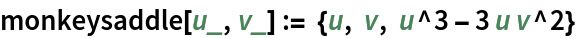In:=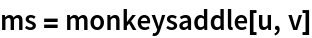Out=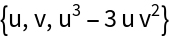Plot the surface:

 In:=Out=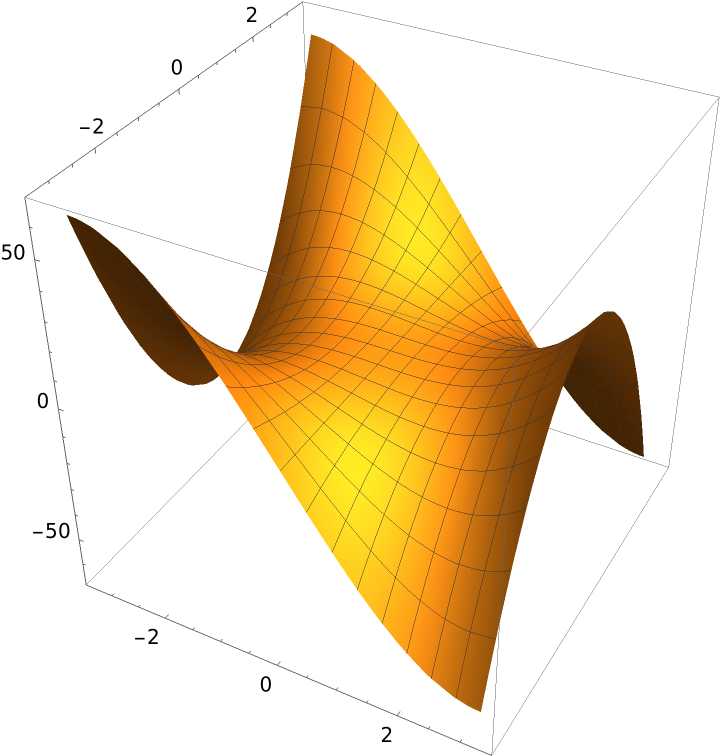The covariant basis:

 In:=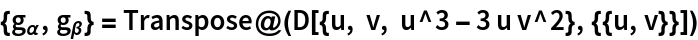Out=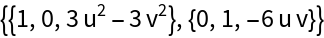The metric tensor:

 In:=Out=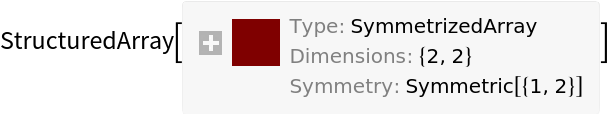The Ricci curvature:

 In:=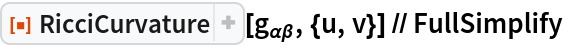Out=### Properties and Relations (2)

Alternatively, Ricci curvature can be computed contracting an index of the Riemann tensor. Compute the same tensor as above:

 In:=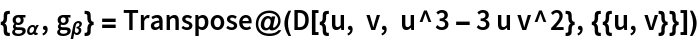Out=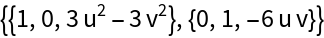In:=Out=The same result is found using the resource functions ArrayContract and RiemannTensor:

 In:=Out=Enrique Zeleny

## Version History

• 1.0.0 – 29 October 2020